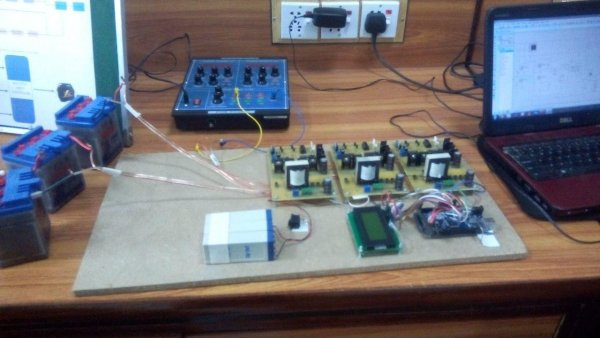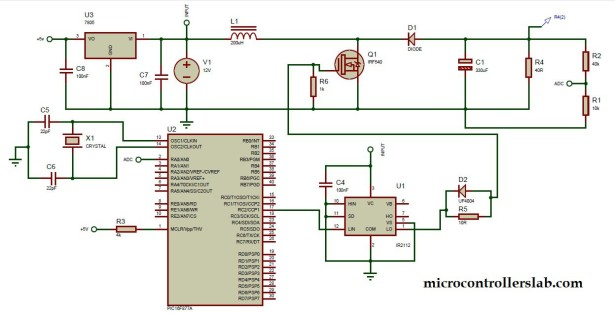# Boost converter using IR2110 and pic microcontroller

Boost converter using IR2110 and pic microcontroller, In this article I am going to post my design of boost converter ? In this article you will learn what is boost converter ? How to design boost converter ? How to use MOSFET as a switch in boost converter and how to drive this MOSFET which is connected in low side configuration. Let’s start this article with article with basic understanding of boost converter and then I will move forward to circuit diagram of boost converter using 1R2210 MOSFET Gate driver and its functionality.

Note : In this article I am just discussing practical aspects to make boost converter . I assume that you know about theoretical calculatons of boost converter design and its basic working. If you don’t know about it I recommend you to go through any power electronics book and read chapter on dc to dc converters.Boost converter is basically a regulator which is used to step up dc voltage without transformer. In other words it is step up transformer for dc voltage. A basic circuit diagram of boost converter is given below.

But this circuit diagram is not full circuit diagram according to practical aspects. Because Switch shown in figure below should be replaced with any semiconductor device like transistor, MOSFET and IGBT. Selection of any one of these components as a switch depends on voltage and current rating of any converter. To use these components we also need driver circuits, For example if you are using MOSFET as a switch, then MOSFET may be used as a low side switch or high side switch that’s why you need a driver circuit. In this article we are using IR2110 MOSFET gate driver IC.

READ  Automatic door lock system using 8051(89c51,89c52) microcontroller

Now I will take one example of boost converter and will show you its circuit diagram which inculdes all practical aspects which you need to make a boost converter. For example we want to make a boost conveter which input voltage is 12V and output voltage is 20 V at 100Khz freuency. The average load current is .5 A . By using these values and with the help of formula’s of boost converter you can easily calculate values of inductor and capacitor. I am not going to discuss it here. Because there are a lot of basics available on power electronics from where you can read method to calculate values of inductor and capacitor. For example we calculated following values :

L = 150uH

C = 33uF

For boost converter formula to calculate duty cycle or in other word on/off time of MOSFET is given below

out put voltage = input voltage / ( 1 – K ) ;   where K is duty cycle

So by putting values of our example

20 = 12 / 1 – k ;

k = .4 or 40 %

So duty cycle of MOSFET is 40 % to get output voltage of  20 V. To get regulated DC output voltage you should use feedback . For example, if input voltage increases, output voltage will also increase according to above given formula of duty cycle. But to maintain output votage constant duty cycle should be reduced equal proportion. For this purpose you need to used microcontroller and you should learn how to used microcontroller for PWM generation and to generate PWM with variable duty cycle. If you want to know about  it check following article :READ  contruction of personal Radar System using PIC MIcrocontroller PIC18f452

### Circuit diagram of boost converter :

Circuit diagram of dc to dc boost converter is given below . Diode D1 shlould be selected according to averaga load current. For example in this example average load current is .5 Ampere. So D1 should have atleast 1 ampere.  Similarly MOSFET should also be selected according to voltage and current rating of your design requirement.

Mosfet is used as a low side configuration . because load is connected to drain of MOSFET. A voltage divider is used to lower voltage to less than 5 volt and then fed to microcontroller ADC pin . Because microcontroller can not read voltage more than 5 volt. This voltage measurement is used to set duty cycle in case of voltage fluctuation at the output. PIC16F877A microcontroller is used to generate PWM.  you should have a knowlege about pic microcontrollers and its programming to write code for PWM and voltage measurement of above circuit diagram. Check our tutorials on pic microcontroller to learn these things. Good luck with your project. Don’t forget to share this with your friends .

For more detail: Boost converter using IR2110 and pic microcontroller

#### Current Project / Post can also be found using:

• pic16f877 boost
• boost circuit using ir2110 driver ic
• boost converter pic